# How to Create Numpy Array in Python

NumPy, which stands for Numerical Python, is a library consisting of multidimensional array objects and the collection of routines for processing those arrays. Using NumPy, mathematical and logical operations on arrays can be performed.

## How to create numpy array

To create a numpy array in Python, use the np.array() functionThe numpy array() accepts either a Python list or a tuple and returns an array.

To demonstrate how to create an array in Python, we will perform all the practicals in Python Jupyter Notebook. Now, you can check your NumPy version using the following code. But first, write the following code inside the Jupyter Notebook cell.

```import numpy as np
print(np.__version__)```

Now run the cell using Ctrl + Enter and see the output.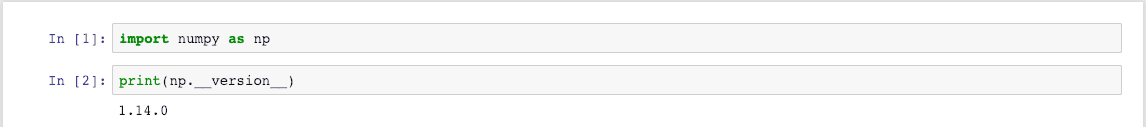Yours might be different as you will see this in the future.

## Create Numpy Array From Python List

To create a numpy array from a Python list, use the np.array() function, pass the list as an argument, and returns an array.

Let’s define a list and then turn that list into the NumPy array.

```app_list = [18, 0, 21, 30, 46]
np_app_list = np.array(app_list)
np_app_list```

First, we defined a List and then turned that list into the NumPy array using the np.array function.

See the output below.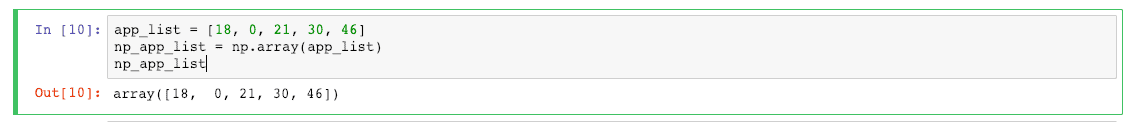Let’s add 5 to all the values inside the numpy array. See the following code.

`np_app_list + 5`

See the following output.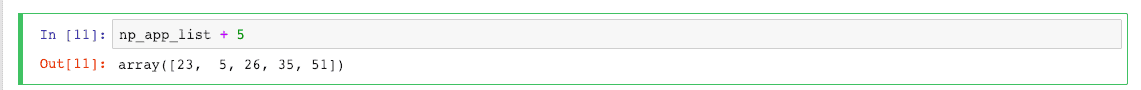## Create Numpy Array From Python Tuple

To create a numpy array from a Python tuple, use the np.array() function, pass the tuple as an argument, and returns an array.

Let’s define a tuple and turn that tuple into an array.

```app_tuple = (18, 19, 21, 30, 46)
np_app_tuple = np.array(app_tuple)
np_app_tuple```

See the output below.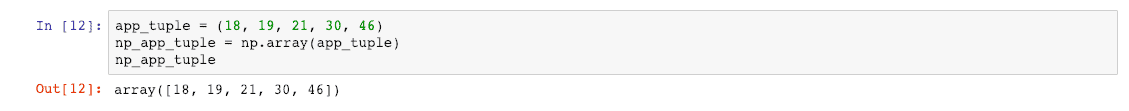Let’s take an example of a complex type in the tuple.

```complex_tuple = (21.00 - 0.j, 19.00 + 9.j, 18.00 + 5.j, 30 - 1.j, 19, 18, 30)
np_complex = np.array(complex_tuple)
np_complex```

We filled the tuple items with complex numbers and some regular integers in the above code.

When we convert the tuple to the numpy arrays, all the items should be converted to the complex numbers in the NumPy array

See the output below.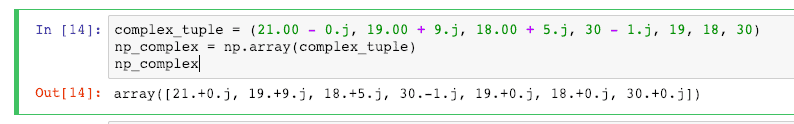You can see that the numpy array items are converted into complex numbers.

The significant difference between a Numpy array and Python Tuple is that if you perform the multiplication operation on the NumPy, all the items in the tuple will be multiplied by a provided integer.

However, that does not make the case with Python Tuple; it will not multiply with each item of the tuple with a provided eight value. Instead, it will create eight times the whole tuple items. See the example below.

See the example of the tuple below.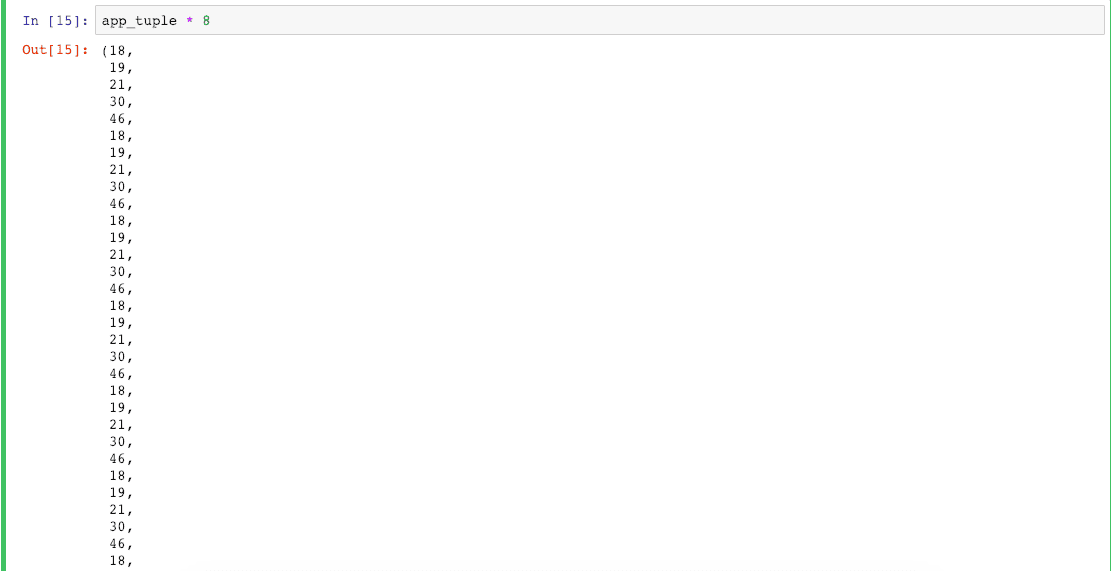You can see that items repeat up to 8 times, but not multiplying 8 with each item.

Now, see the case with NumPy arrays.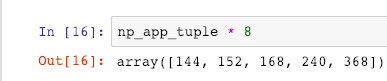See, eight is multiplied with each value of the NumPy array.

That’s it.

## Related posts

Numpy array to list

Python array length

Python array contains

This site uses Akismet to reduce spam. Learn how your comment data is processed.